# GMAT Math : Yes/No Data Sufficiency

## Example Questions

### Example Question #1 : Yes/No Data Sufficiency

Is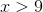?

1.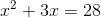2.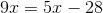Statement (1) ALONE is sufficient, but statement (2) alone is not sufficient to answer the question asked

Statement (2) ALONE is sufficient, but statement (1) alone is not sufficient to answer the question asked

Statements (1) and (2) TOGETHER are NOT sufficient to answer the question asked, and additional data specific to the problem are needed

Both statements (1) and (2) TOGETHER are sufficient to answer the question asked; but NEITHER statement ALONE is sufficient

Explanation:

Importantly, this is a Yes/No Data Sufficiency question. In such a question, even if there are multiple solutions for a variable you will have sufficient information if all solutions provide the same answer ("always Yes" or "always No"). For this reason, it is always important to note whether you're dealing with a "what is the value?" question or a "Yes/No" question.

Here you're asked whether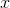is greater than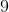With statement 1, you're provided with a quadratic. But even though quadratics generally yield multiple solutions, note that a quadratic as a statement can provide sufficient information if:

-All solutions provide the same answer (as you'll see here)

-It is a "special" quadratic that factors to only one solution (in the form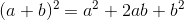or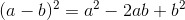)

For this reason, you should make a point of always doing (or at least beginning) the math on quadratics to see what the quadratic will yield. Here you can start by subtracting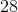from both sides to yield: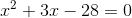And then factor to: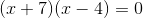When you solve, by setting each parenthetical equal to zero, you'll have: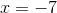or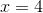Note that each possible value for x is less than 9, meaning that each gives the answer "no." So while you don't know exactly what x is, you do have sufficient information to determine that the answer to the question is "no," so statement 1 is sufficient. Consequently you can eliminate choices "Statement (2) ALONE is sufficient, but statement (1) alone is not sufficient to answer the question asked", "Both statements (1) and (2) TOGETHER are sufficient to answer the question asked; but NEITHER statement ALONE is sufficient", and Statements (1) and (2) TOGETHER are NOT sufficient to answer the question asked, and additional data specific to the problem are needed"

With statement 2, savvy examinees will note that with a linear equation there can be only one solution. Here you can save time - if you know that the equation will yield exactly one value, then that exact value will guarantee exactly one answer to the overall question (that value will either be greater than 9, so "yes," or it will not, so "no"). Therefore this statement must be sufficient, yielding the answer "EACH statement ALONE is sufficient to answer the question asked"

Of course, if you do not see that at first, there is no harm in doing the math. If you subtract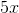from each side ofyou will have: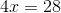Then dividing both sides by 4 will yield, guaranteeing the answer "no."

Note here, also, that your job is only to determine whether the information is sufficient to answer the question, not whether that answer happens to be "yes." So a consistent, guaranteed "no" answer means that the information is "sufficient."

### Example Question #1 : Yes/No Data Sufficiency

In a retail store, the average (arithmetic mean) sale for month M was d dollars. Was the average (arithmetic mean) sale for month J at least 20 percent higher than that for month M?

1. For month M, total revenue from sales was $3,500. 2. For month J, total revenue from sales was$6,000

Statement (1) ALONE is sufficient, but statement (2) alone is not sufficient to answer the question asked

Statements (1) and (2) TOGETHER are NOT sufficient to answer the question asked, and additional data specific to the problem are needed

Both statements (1) and (2) TOGETHER are sufficient to answer the question asked; but NEITHER statement ALONE is sufficient

Statement (2) ALONE is sufficient, but statement (1) alone is not sufficient to answer the question asked

Statements (1) and (2) TOGETHER are NOT sufficient to answer the question asked, and additional data specific to the problem are needed

Explanation:

This problem is a classic example of how the GMAT tests "precision in language." The question asks about the average sale in each month, but the data you're given is about the total revenue from sales. And of course total revenue comes from adding up all of the individual sales in a month. The average sale would be calculated as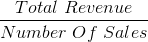As you assess the statements, it should be clear that neither is sufficient alone; each tells you only about one of the two months, leaving the other month completely undefined.

And even when you take them together, note that you have all the total sales revenue information you need for the numerators of the average sale calculation, but none of the "number of sales" information necessary to assess the exact question being asked. Therefore you do not have sufficient information to answer the question, and the correct answer is "Statements (1) and (2) TOGETHER are NOT sufficient to answer the question asked, and additional data specific to the problem are needed". The lesson? Pay close attention to the specific question being asked; as you can see from the statistics, those who miss this problem pick "Both statements (1) and (2) TOGETHER are sufficient to answer the question asked; but NEITHER statement ALONE is sufficient", almost always because they compare "Month M to Month J" but along the total revenue metric, when that's not what the question asks.

### Example Question #1 : Yes/No Data Sufficiency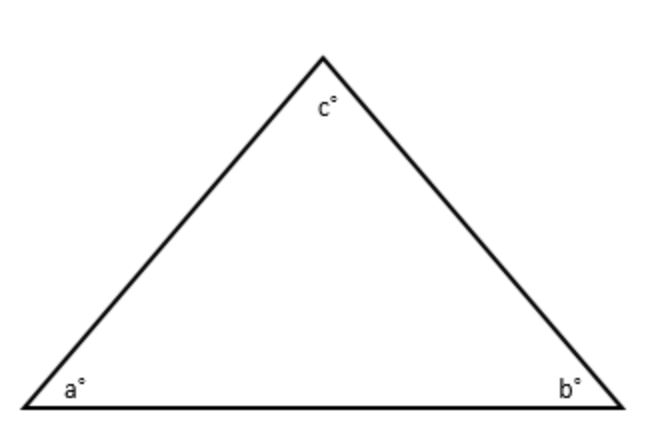(NOTE: not drawn to scale)

Is a > 85?

(1) b > 75

(2) c > 95

Statements (1) and (2) TOGETHER are NOT sufficient to answer the question asked, and additional data specific to the problem are needed

Statement (1) ALONE is sufficient, but statement (2) alone is not sufficient to answer the question asked

Statement (2) ALONE is sufficient, but statement (1) alone is not sufficient to answer the question asked

Both statements (1) and (2) TOGETHER are sufficient to answer the question asked; but NEITHER statement ALONE is sufficient

Statement (2) ALONE is sufficient, but statement (1) alone is not sufficient to answer the question asked

Explanation:

This problem rewards those who leverage all assets in a problem. Because of the yes/no format, you do not need to know the exact measure of angle a (or any angle in the triangle) as long as you can prove that a is either always greater than 85 ("yes") or always less than (or equal to) 85 ("no").

Statement 1 is not sufficient, as it allows for each possibility of yes and no. But statement 2 is sufficient. Note that all angles in a triangle must equal 180. So if c is greater than 95, then a + b must be less than 85 in order for the total sum to be 180. Since a and b cannot be negative, they each must be less than 85, meaning that the answer is a definite "no." Therefore statement 2 is sufficient and the correct answer is "Statement (2) ALONE is sufficient, but statement (1) alone is not sufficient to answer the question asked".

### Example Question #4 : Yes/No Data Sufficiency

Is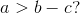1.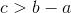2.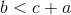Both statements (1) and (2) TOGETHER are sufficient to answer the question asked; but NEITHER statement ALONE is sufficient

Statement (2) ALONE is sufficient, but statement (1) alone is not sufficient to answer the question asked

Statement (1) ALONE is sufficient, but statement (2) alone is not sufficient to answer the question asked

Statements (1) and (2) TOGETHER are NOT sufficient to answer the question asked, and additional data specific to the problem are needed

Explanation:

When Yes/No questions ask whether a certain algebraic expression is true, as you see here where the question asks about an algebraic inequality, it is generally most efficient to try to make the given statements look like the question. If you can effectively "mirror" the question with the statements, you can prove sufficiency.

For example, with statement 1, you can take the given information:And try to make it look like the question, which has a positive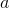on one side of the inequality and the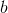andterms on the other. To accomplish that, addto each side: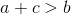And then subtract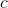from each side: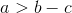This statement then directly answers the question, so the answer must be "yes." Statement 1 is therefore sufficient.

You can work on Statement 2 the same way. If you take the given statement,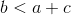, and subtractfrom each side, you'll again have mirrored the question: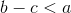This also proves that the answer is "yes" and is therefore sufficient information. The answer, then, is "EACH statement ALONE is sufficient to answer the question asked".

### Example Question #5 : Yes/No Data Sufficiency

Is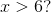1.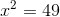2.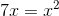Statement (2) ALONE is sufficient, but statement (1) alone is not sufficient to answer the question asked

Statement (1) ALONE is sufficient, but statement (2) alone is not sufficient to answer the question asked

Both statements (1) and (2) TOGETHER are sufficient to answer the question asked; but NEITHER statement ALONE is sufficient

Statements (1) and (2) TOGETHER are NOT sufficient to answer the question asked, and additional data specific to the problem are needed

Both statements (1) and (2) TOGETHER are sufficient to answer the question asked; but NEITHER statement ALONE is sufficient

Explanation:

This Yes/No Data Sufficiency problem rewards those who play devil's advocate. Each statement screamscould be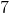! (which would give the answer "yes,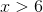but each allows for an alternative value of(each of which would give the answer "no" to the question "is).

With statement 1, it is important to recognize thatallows for two answers: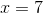and. Remember: although on a day to day basis you think about positive numbers much more often than you think about negatives, you must always consider the possibility of negative values on the GMAT! Since each of these values provides a different answer to the main question (7 is greater than 6 but -7 is less than 6), statement 1 is not sufficient, so you can eliminate answer choices "Statement (1) ALONE is sufficient, but statement (2) alone is not sufficient to answer the question asked" and "Statements (1) and (2) TOGETHER are NOT sufficient to answer the question asked, and additional data specific to the problem are needed".

Statement 2 also allows for different values of. While you might be tempted to just divide both sides by, note that you cannot do so unless you know for certain that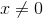. Dividing by 0 is not allowed, so ifcould be 0 you have to treat this problem like a quadratic and solve that way. If you do so, you will subtract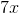from both sides of the given equation, leaving: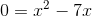Then you can factor out the commonterm: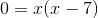And then solve: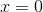or. Since each gives a different answer to the overall question, this statement is also insufficient and you can eliminate choice "Statement (2) ALONE is sufficient, but statement (1) alone is not sufficient to answer the question asked".

When you combine the statements, you know from statement 1 thatmust be either -7 or 7 and from statement 2 thatmust be 0 or 7. Sinceis the only possible solution consistent with both statements, you have a concrete value forand can definitively answer the question: "yes,." This is sufficient information, so the correct answer is " Both statements (1) and (2) TOGETHER are sufficient to answer the question asked; but NEITHER statement ALONE is sufficient".

### Example Question #6 : Yes/No Data Sufficiency

Is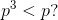1.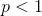2.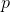is an integer

Statement (2) ALONE is sufficient, but statement (1) alone is not sufficient to answer the question asked

Statement (1) ALONE is sufficient, but statement (2) alone is not sufficient to answer the question asked

Statements (1) and (2) TOGETHER are NOT sufficient to answer the question asked, and additional data specific to the problem are needed

Both statements (1) and (2) TOGETHER are sufficient to answer the question asked; but NEITHER statement ALONE is sufficient

Statements (1) and (2) TOGETHER are NOT sufficient to answer the question asked, and additional data specific to the problem are needed

Explanation:

This problem rewards students who remember to “Play Devil’s Advocate” when picking numbers. Remember to examine your assumptions around the numbers you have picked and test the boundaries of the problem. Statement (1) gives that. If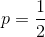, then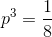Since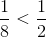, this gives an answer of “Yes” to the question posed.

However, consider:could equal 0, in which case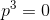This would mean that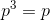, giving you an answer of “No” to the question posed.

Because you can get both a “Yes” and a “No”, statement (1) is insufficient, eliminating "Statement (1) ALONE is sufficient, but statement (2) alone is not sufficient to answer the question asked" and "EACH statement ALONE is sufficient to answer the question asked".

Statement (2) states thatis an integer. This means thatcould be equal to -2 , in which case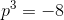. This would give you an answer of “Yes” to the question posed. However, sincecould also be 0, using the work you did for statement (1) shows that you can also get a “no” for the information given. Since statement (2) allows for multiple answers to the question posed, you must conclude that statement (2) is insufficient and eliminate "Statement (2) ALONE is sufficient, but statement (1) alone is not sufficient to answer the question asked".

Taking both statements together, you should recognize that the two statements together are still insufficient based on the work you have already done. The numbers chosen to test statement (2) also were within the boundaries established by statement (1). Since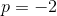and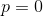fit both statements and give an answer of “Yes” and “No” respectively, you can conclude that statements (1) and (2) are not sufficient even when taken together, so you must eliminate "Both statements (1) and (2) TOGETHER are sufficient to answer the question asked; but NEITHER statement ALONE is sufficient" and choose answer choice "Statements (1) and (2) TOGETHER are NOT sufficient to answer the question asked, and additional data specific to the problem are needed".

### Example Question #1321 : Data Sufficiency Questions

Is the area of circular region X greater than the area of circular region Y?

1. The circumference of circle X is greater than the circumference of circle Z, and the circumference of circle Z is less than the circumference of circle Y.
2. The radius of circle X is greater than the radius of circle Y.

Statement (2) ALONE is sufficient, but statement (1) alone is not sufficient to answer the question asked

Both statements (1) and (2) TOGETHER are sufficient to answer the question asked; but NEITHER statement ALONE is sufficient

Statements (1) and (2) TOGETHER are NOT sufficient to answer the question asked, and additional data specific to the problem are needed

Statement (1) ALONE is sufficient, but statement (2) alone is not sufficient to answer the question asked

Statement (2) ALONE is sufficient, but statement (1) alone is not sufficient to answer the question asked

Explanation:

This is a “yes/no” question that asks you to determine whether circular region X has a larger area than does circular region Y. Because statement 2 is much less wordy, it may be easier to evaluate, so you should start there.

Statement 2: This statement gives you the relationship between the radius of circle X and that of circle Y. Circle X has the larger radius. Since the formula for the area of a circle,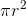, is a constant, it is true that a larger radius definitely means a larger area of the circle.

If you need to prove this to yourself, remember that you can quickly do this by picking numbers.If circles X and Y had radii of 4 and 3 respectively, they would have areas of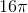and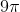. A quick look at the equation for the area of a circle should confirm that the relationship between these two will remain constant as long as the radius of circle X is larger than that of circle Y, so statement 2 is sufficient. Eliminate "Statement (1) ALONE is sufficient, but statement (2) alone is not sufficient to answer the question asked", "Both statements (1) and (2) TOGETHER are sufficient to answer the question asked; but NEITHER statement ALONE is sufficient", and "Statements (1) and (2) TOGETHER are NOT sufficient to answer the question asked, and additional data specific to the problem are needed".

Statement 1: This statement compares the circumference of circles X and Y with that of circle Z. The statement can be rewritten as “circumference X > circumference Z” and “circumference Y > circumference Z.” However, this does not allow for a comparison of circles x and y; it simply indicates that each are larger than circle Z.

Remember that you can often prove a statement insufficient by Playing Devil's Advocate and picking numbers even if the wording seems to make it abstract. Notice that if circle Z had a radius of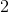and a circumference of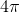, that as long as X and Y had larger radii, they would fulfill the constraints of the statement. Both could have the same radius (for instance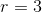) and have the same circumference, or one could be bigger than the other. Since there is no way of knowing, this information is not sufficient. Eliminate "EACH statement ALONE is sufficient to answer the question asked".

The correct answer is "Statement (2) ALONE is sufficient, but statement (1) alone is not sufficient to answer the question asked".

### Example Question #1322 : Data Sufficiency Questions

An artist's portfolio consisting of 1,000 photos is divided into 20 subjects. After an extensive photo shoot, two more subjects are then added to the portfolio. Is the average (arithmetic mean) number of photos in each subject greater than 55?

1. Each of the new subjects has fewer than 117 photos.
2. Each of the new subjects has more than 71 photos.

Statements (1) and (2) TOGETHER are NOT sufficient to answer the question asked, and additional data specific to the problem are needed

Statement (1) ALONE is sufficient, but statement (2) alone is not sufficient to answer the question asked

Both statements (1) and (2) TOGETHER are sufficient to answer the question asked; but NEITHER statement ALONE is sufficient

Statement (2) ALONE is sufficient, but statement (1) alone is not sufficient to answer the question asked

Statements (1) and (2) TOGETHER are NOT sufficient to answer the question asked, and additional data specific to the problem are needed

Explanation:

From the question stem, the average of the current portfolio is 50 photos (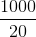). Statement (1) indicates that each of the two new subjects has less than 117 photos. At most, each of the two new subjects would contain 116 photos. This would bring the new average to a maximum 56 photos (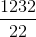). While this is the maximum, there could always be fewer photos. This allows for both greater than 55 photos on average and fewer than 55 photos on average. Since this statement yields both "yes" and "no" as a response, statement (1) is not sufficient. Statement (2 tells us that each new subject has more than 71 photos. At least, each of the two new subjects would contain 72 photos. This means that the new average must be at least 52 photos (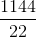). Of course, this also allows for both greater than 55 and fewer than 55 photos on average, so statement (2) is not sufficient. Taking the two statements together, we know that the new average is between 52 and 56; however this is still not sufficient to say whether the average is greater than 55 or not. The two statements together are not sufficient and the answer is accordingly E. Tip: A quicker way to evaluate the statements is to maintain the average of 50 first, and then see whether there are enough additional photos to push the average to 55. For statement (1), subtract 50 photos from the maximum possible of 116. That 50 subtracted is enough to maintain the average; so at this point, we have an average of 50 with an additional 66 photos per subject. Since we have 2 new subjects, we have 132 additional photos (66 * 2) over 22 subjects to try and push the average over 55. If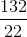yields a number larger than 5, then the total average will be greater than 55. This technique requires much easier calculating than dividing 1232 by 22. Repeat the same procedure for statement (2).

Tired of practice problems?

Try live online GMAT prep today.